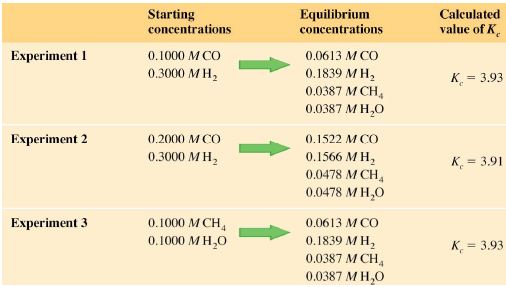Chemistry

# Obtaining Equilibrium Constant Expressions

Equilibrium concentrations for a reaction cannot be predicted theoretically. They must be obtained experimentally and then substituted into the equilibrium constant expression in order to calculate a value for Kc.

Example

Consider the reaction below:

CO (g) + 3H2 = CH4 (g) + H2O (g)

A series of experiments was done, starting with different concentrations and proportions of the reactants as shown in the table below.Experiments 1 and 2 start with the reactants; whereas experiment 3 starts with the products. The equilibrium-constant expression for this reaction is:

Kc = {[CH4] x [H2O]} / {[CO] x [H2]3}

If we substitute the equilibrium concentrations for any of the experiments as per the figures from the table above, we obtain the same ratio. For example, from experiment I we can calculate the following Kc:

Kc = (0.0387 x 0.0387) / (0.0613 x 0.18393)

Kc = 3.93

Regardless of the initial concentrations (whether they be reactants or products), the law of mass action means that the reaction will always settle into an equilibrium where the equilibrium-constant expression equals Kc.